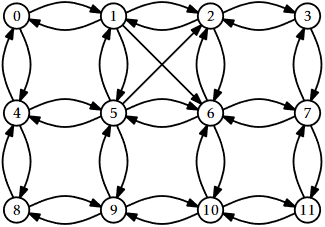# 6.2: A Graph as a Collection of Lists

$$\newcommand{\vecs}{\overset { \rightharpoonup} {\mathbf{#1}} }$$ $$\newcommand{\vecd}{\overset{-\!-\!\rightharpoonup}{\vphantom{a}\smash {#1}}}$$$$\newcommand{\id}{\mathrm{id}}$$ $$\newcommand{\Span}{\mathrm{span}}$$ $$\newcommand{\kernel}{\mathrm{null}\,}$$ $$\newcommand{\range}{\mathrm{range}\,}$$ $$\newcommand{\RealPart}{\mathrm{Re}}$$ $$\newcommand{\ImaginaryPart}{\mathrm{Im}}$$ $$\newcommand{\Argument}{\mathrm{Arg}}$$ $$\newcommand{\norm}{\| #1 \|}$$ $$\newcommand{\inner}{\langle #1, #2 \rangle}$$ $$\newcommand{\Span}{\mathrm{span}}$$ $$\newcommand{\id}{\mathrm{id}}$$ $$\newcommand{\Span}{\mathrm{span}}$$ $$\newcommand{\kernel}{\mathrm{null}\,}$$ $$\newcommand{\range}{\mathrm{range}\,}$$ $$\newcommand{\RealPart}{\mathrm{Re}}$$ $$\newcommand{\ImaginaryPart}{\mathrm{Im}}$$ $$\newcommand{\Argument}{\mathrm{Arg}}$$ $$\newcommand{\norm}{\| #1 \|}$$ $$\newcommand{\inner}{\langle #1, #2 \rangle}$$ $$\newcommand{\Span}{\mathrm{span}}$$

Adjacency list representations of graphs take a more vertex-centric approach. There are many possible implementations of adjacency lists. In this section, we present a simple one. At the end of the section, we discuss different possibilities. In an adjacency list representation, the graph $$G=(V,E)$$ is represented as an array, $$\mathtt{adj}$$, of lists. The list $$\mathtt{adj[i]}$$ contains a list of all the vertices adjacent to vertex $$\mathtt{i}$$. That is, it contains every index $$\mathtt{j}$$ such that $$\mathtt{(i,j)}\in E$$.

    int n;
n = n0;
for (int i = 0; i < n; i++)
}


(An example is shown in Figure $$\PageIndex{1}$$.) In this particular implementation, we represent each list in $$\mathtt{adj}$$ as an ArrayStack, because we would like constant time access by position. Other options are also possible. Specifically, we could have implemented $$\mathtt{adj}$$ as a DLList.Figure $$\PageIndex{1}$$: A graph and its adjacency lists

The $$\mathtt{addEdge(i,j)}$$ operation just appends the value $$\mathtt{j}$$ to the list $$\mathtt{adj[i]}$$:

    void addEdge(int i, int j) {
}


This takes constant time.

The $$\mathtt{removeEdge(i,j)}$$ operation searches through the list $$\mathtt{adj[i]}$$ until it finds $$\mathtt{j}$$ and then removes it:

    void removeEdge(int i, int j) {
while (it.hasNext()) {
if (it.next() == j) {
it.remove();
return;
}
}
}


This takes $$O(\deg(\mathtt{i}))$$ time, where $$\deg(\mathtt{i})$$ (the degree of $$\mathtt{i}$$) counts the number of edges in $$E$$ that have $$\mathtt{i}$$ as their source.

The $$\mathtt{hasEdge(i,j)}$$ operation is similar; it searches through the list $$\mathtt{adj[i]}$$ until it finds $$\mathtt{j}$$ (and returns true), or reaches the end of the list (and returns false):

    boolean hasEdge(int i, int j) {
}


This also takes $$O(\deg(\mathtt{i}))$$ time.

The $$\mathtt{outEdges(i)}$$ operation is very simple; it returns the list $$\mathtt{adj[i]}$$ :

    List<Integer> outEdges(int i) {
}


This clearly takes constant time.

The $$\mathtt{inEdges(i)}$$ operation is much more work. It scans over every vertex $$j$$ checking if the edge $$\mathtt{(i,j)}$$ exists and, if so, adding $$\mathtt{j}$$ to the output list:

    List<Integer> inEdges(int i) {
List<Integer> edges = new ArrayStack<Integer>(Integer.class);
for (int j = 0; j < n; j++)
return edges;
}


This operation is very slow. It scans the adjacency list of every vertex, so it takes $$O(\mathtt{n} + \mathtt{m})$$ time.

The following theorem summarizes the performance of the above data structure:

Theorem $$\PageIndex{1}$$

The AdjacencyLists data structure implements the Graph interface. An AdjacencyLists supports the operations

• $$\mathtt{addEdge(i,j)}$$ in constant time per operation;
• $$\mathtt{removeEdge(i,j)}$$ and $$\mathtt{hasEdge(i,j)}$$ in $$O(\deg(\mathtt{i}))$$ time per operation;
• $$\mathtt{outEdges(i)}$$ in constant time per operation; and
• $$\mathtt{inEdges(i)}$$ in $$O(\mathtt{n}+\mathtt{m})$$ time per operation.

The space used by a AdjacencyLists is $$O(\mathtt{n}+\mathtt{m})$$.

As alluded to earlier, there are many different choices to be made when implementing a graph as an adjacency list. Some questions that come up include:

• What type of collection should be used to store each element of $$\mathtt{adj}$$? One could use an array-based list, a linked-list, or even a hashtable.
• Should there be a second adjacency list, $$\mathtt{inadj}$$, that stores, for each $$\mathtt{i}$$, the list of vertices, $$\mathtt{j}$$, such that $$\mathtt{(j,i)}\in E$$? This can greatly reduce the running-time of the $$\mathtt{inEdges(i)}$$ operation, but requires slightly more work when adding or removing edges.
• Should the entry for the edge $$\mathtt{(i,j)}$$ in $$\mathtt{adj[i]}$$ be linked by a reference to the corresponding entry in $$\mathtt{inadj[j]}$$?
• Should edges be first-class objects with their own associated data? In this way, $$\mathtt{adj}$$ would contain lists of edges rather than lists of vertices (integers).

Most of these questions come down to a tradeoff between complexity (and space) of implementation and performance features of the implementation.

6.2: A Graph as a Collection of Lists is shared under a CC BY license and was authored, remixed, and/or curated by LibreTexts.